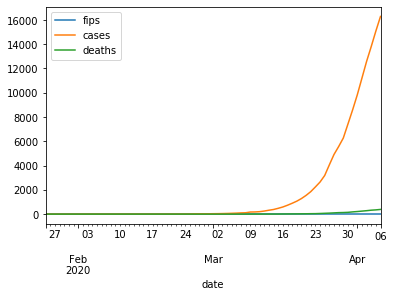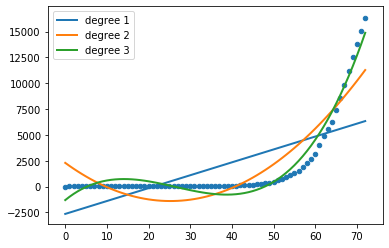# Polynomial Interpolation Using Python Pandas, Numpy And Sklearn

In this post, We will use covid 19 data to go over polynomial interpolation.

Before we delve in to our example, Let us first import the necessary package pandas.

In :
import pandas as pd
from matplotlib import pyplot as plt
import numpy as np

In :
df=pd.read_csv('covid19_us_states.csv',encoding='UTF-8')


df is a datraframe which contains time series covid 19 data for all US states. Let us take a peak in to the data for California.

In :
df[df.state=='California'].head(2)

Out:
date state fips cases deaths
5 1/25/2020 California 6 1 0
9 1/26/2020 California 6 2 0

Let us covert the date in to Python datetime object and set the index as date.

In :
df['date'] = pd.to_datetime(df['date'])

In :
df.set_index('date',inplace=True)


Let us do a line plot for covid 19 cases of California.

In :
df[df.state=='California'].plot.line()

Out:
<matplotlib.axes._subplots.AxesSubplot at 0x7fd51f6eea90>## Polynomial Interpolation Using Sklearn

We would need Ridge, PolynomialFeatures and make_pipeline to find the right polynomial to fit the covid 19 California data.

Ridge is a l2 regularization technique. PolynomialFeatures generates polynomial and interaction features. make_pipeline is a function to build the pipeline.

In :
from sklearn.linear_model import Ridge
from sklearn.preprocessing import PolynomialFeatures
from sklearn.pipeline import make_pipeline

In :
X = np.array(range(len(df[df.state=='California'].index))).reshape(-1,1)
y = df[df.state=='California']['cases']
models = []
for count, degree in enumerate([1, 2, 3]):
model = make_pipeline(PolynomialFeatures(degree), Ridge(alpha=0.001))
model.fit(X, y)
models.append(model)
y_pred = model.predict(X)
plt.plot(X, y_pred, linewidth=2, label='degree %d' % degree)
plt.legend(loc='upper left')
plt.scatter(X, y, s=20, marker='o', label='training points')
plt.show()In the above code, we can see polynomials of degree 1, 2 and 3. As we see polynomial of degree 3 matches very close to the real data.